#1. 云栖社区>
2. 博客>
3. 正文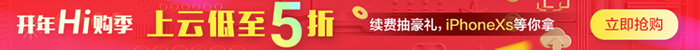## 机器学习笔记(3)：多类逻辑回归``` 1 from mxnet import gluon
2 from mxnet import ndarray as nd
3 import matplotlib.pyplot as plt
4 import mxnet as mx
5 from mxnet import autograd
6
7 def transform(data, label):
8     return data.astype('float32')/255, label.astype('float32')
9
10 #训练数据集（需联网下载，网速慢时，会很卡）
11 mnist_train = gluon.data.vision.FashionMNIST(train=True, transform=transform)
12
13 #测试数据集（需联网下载）
14 mnist_test = gluon.data.vision.FashionMNIST(train=False, transform=transform)
15
16 # data, label = mnist_train
17 # ('example shape: ', data.shape, 'label:', label)
18
19 #显示服饰图片
20 def show_images(images):
21     n = images.shape
22     _, figs = plt.subplots(1, n, figsize=(15, 15))
23     for i in range(n):
24         figs[i].imshow(images[i].reshape((28, 28)).asnumpy())
25         figs[i].axes.get_xaxis().set_visible(False)
26         figs[i].axes.get_yaxis().set_visible(False)
27     plt.show()
28
29 #获取图片对应分类标签文本
30 def get_text_labels(label):
31     text_labels = [
32         'T 恤', '长 裤', '套头衫', '裙 子', '外 套',
33         '凉 鞋', '衬 衣', '运动鞋', '包 包', '短 靴'
34     ]
35     return [text_labels[int(i)] for i in label]
36
37 #下面这些代码，用于辅助大家理解示例图片数据集内部结构
38 # tup1 = mnist_train[0:1] #取出训练集的第1个样本
39 # print(type(tup1)) #<class 'tuple'> 可以看出这是个元组类型
40 # print(len(tup1)) #2 有2个元素
41 # print(type(tup1)) #<class 'mxnet.ndarray.ndarray.NDArray'> 第1个元素是一个矩阵
42 # print(type(tup1)) #<class 'numpy.ndarray'> 第2个元素是numpy的矩阵
43 # print(tup1.shape) #(1, 28, 28, 1) 第1个元素是一个四维矩阵，用来存储每张图中的像素点对应的值,最后1维表示RGB通道，这里只取了1个通道
44 # print(tup1.shape) #(1,) 第2个元素用于表示图片对应的文本分类的索引值
45 # print(tup1) #打印第1个元素（即：四维矩阵的值），<NDArray 1x28x28x1 @cpu(0)> 结果太长，就不列在注释里了
46 # print(tup1) #[2.]，打印第2个元素（即：该图片对应的分类索引数值）
47 # print(get_text_labels(tup1)) #显示分类索引值对应的文本['pullover']
48
49 #取出训练集中的图片数据，以及图片标签索引值
50 data, label = mnist_train[0:10]
51
52 #打印数据集的相关信息
53 print('example shape: ', data.shape, 'label:', label)
54
55 #显示图片
56 show_images(data)
57
58 #打印图片分类标签
59 print(get_text_labels(label))```
View Code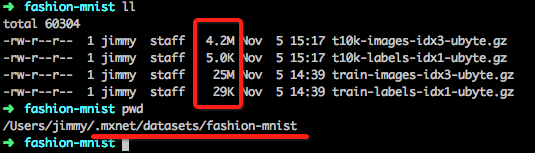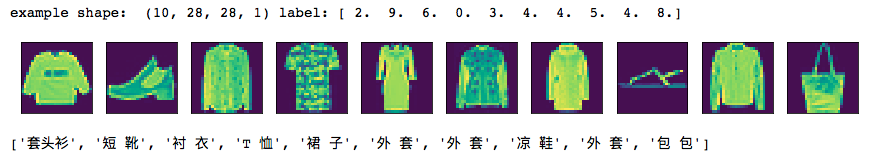``` 1 #批量读取数据
2 batch_size = 256
3 #训练集
4 train_data = gluon.data.DataLoader(mnist_train, batch_size, shuffle=True)
5 #测试集
6 test_data = gluon.data.DataLoader(mnist_test, batch_size, shuffle=False)
7
8 #每张图片的像素用向量表示，就是28*28的长度，即：784
9 num_inputs = 784
10 #要预测10张图片，即：输出结果长度为10的向量
11 num_outputs = 10
12
13 #初始化权重W、偏置b参数矩阵
14 W = nd.random_normal(shape=(num_inputs, num_outputs))
15 b = nd.random_normal(shape=num_outputs)
16
17 params = [W, b]
18
19 #附加梯度，方便后面用梯度下降法计算
20 for param in params:
View Code``` 1 #归一化函数
2 def softmax(X):
3     exp = nd.exp(X)
4     partition = exp.sum(axis=1, keepdims=True)
5     return exp / partition
6
7 #计算模型(仍然是类似y=w.x+b的方程)
8 def net(X):
9     return softmax(nd.dot(X.reshape((-1, num_inputs)), W) + b)
10
11 #损失函数(使用交叉熵函数)
12 def cross_entropy(yhat, y):
13     return - nd.pick(nd.log(yhat), y)
14
15 #梯度下降法
16 def SGD(params, lr):
17     for param in params:
18         param[:] = param - lr * param.grad```
View Code``` 1 #计算准确度
2 def accuracy(output, label):
3     return nd.mean(output.argmax(axis=1) == label).asscalar()
4
5 def _get_batch(batch):
6     if isinstance(batch, mx.io.DataBatch):
7         data = batch.data
8         label = batch.label
9     else:
10         data, label = batch
11     return data, label
12
13 #评估准确度
14 def evaluate_accuracy(data_iterator, net):
15     acc = 0.
16     if isinstance(data_iterator, mx.io.MXDataIter):
17         data_iterator.reset()
18     for i, batch in enumerate(data_iterator):
19         data, label = _get_batch(batch)
20         output = net(data)
21         acc += accuracy(output, label)
22     return acc / (i+1)```
View Code

mean类似sql中的avg函数，就是求平均值，即把一个矩阵的所有元数加起来，然后除以元数个数

```from mxnet import ndarray as nd
x = nd.array([1,2,3,4,5,6]);
print(x,x.mean(),(1+2+3+4+5+6)/6.0)
```

```[ 1.  2.  3.  4.  5.  6.]
<NDArray 6 @cpu(0)>
[ 3.5]
<NDArray 1 @cpu(0)> 3.5```

```from mxnet import ndarray as nd
x = nd.array([1,4,7,3,6])
print(x.argmax(axis=0))
```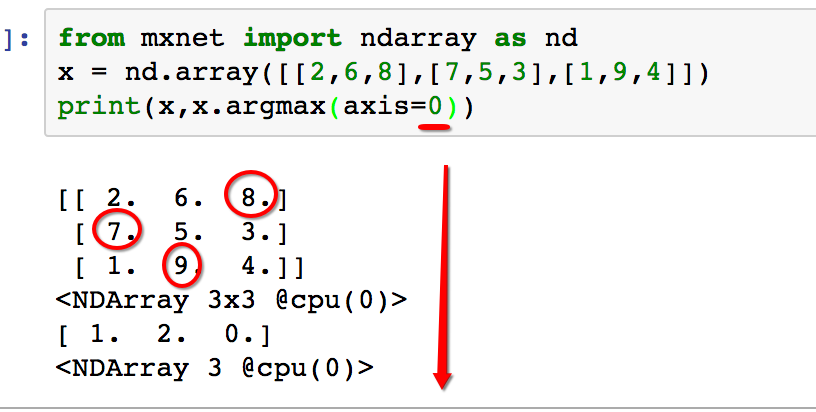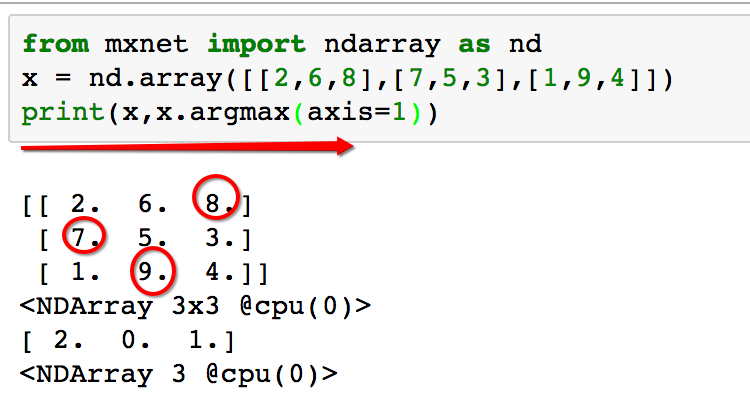axis为1时，输出的索引，为列下标（即：第几列），显然8在第2列，7在第0列，9在第1列。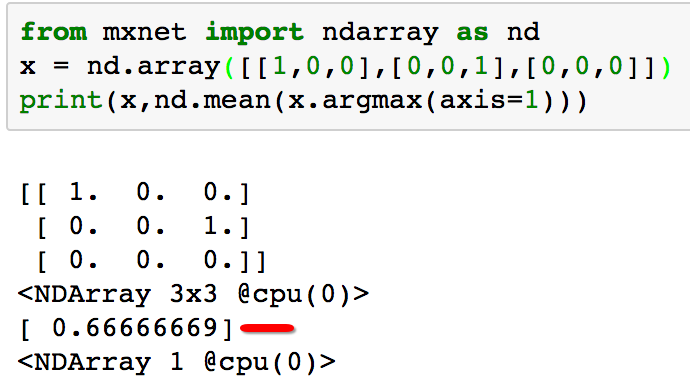``` 1 #学习率
2 learning_rate = .1
3
4 #开始训练
5 for epoch in range(5):
6     train_loss = 0.
7     train_acc = 0.
8     for data, label in train_data:
10             output = net(data)
11             loss = cross_entropy(output, label)
12         loss.backward()
13         SGD(params, learning_rate / batch_size)
14         train_loss += nd.mean(loss).asscalar()
15         train_acc += accuracy(output, label)
16
17     test_acc = evaluate_accuracy(test_data, net)
18     print("Epoch %d. Loss: %f, Train acc %f, Test acc %f" % (
19         epoch, train_loss / len(train_data), train_acc / len(train_data), test_acc))```
View Code```1 #显示结果
2 data, label = mnist_test[0:10]
3 show_images(data)
4 print('true labels')
5 print(get_text_labels(label))
6
7 predicted_labels = net(data).argmax(axis=1)
8 print('predicted labels')
9 print(get_text_labels(predicted_labels.asnumpy()))```
View Code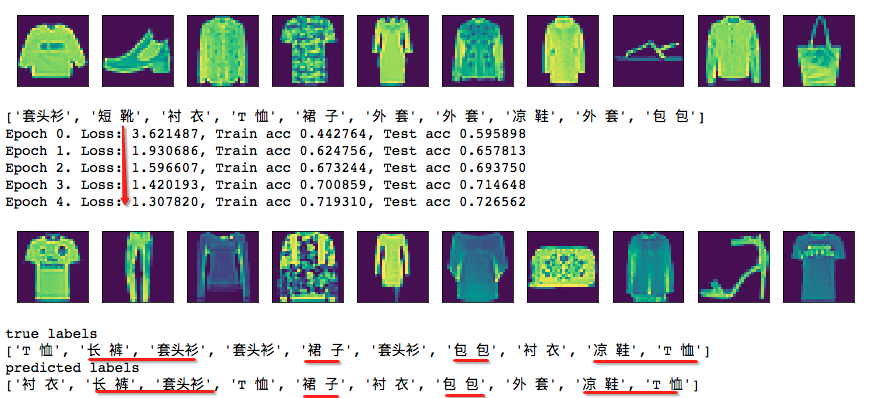【云栖快讯】一站式开发者服务，海量学习资源免费学  详情请点击

+ 关注